Updated LETG Higher Order EfficienciesUpdated LETG Higher Order Efficiencies

April 2011

Summary of Results

Using LETG/ACIS-S spectra from 6 observations of bright continuum sources, relative grating efficiencies (normalized to 1st order) were measured for m = 2 to 10 for < 80 Å. In the worst case, near 12 Å, measured efficiencies were consistently found to be 15-20% smaller than the N0006 GREFFs for orders m = 3-6, with all orders' efficiencies at least slightly smaller than the model over most of the measured wavelength range, with some exceptions for 2nd and 3rd order. Calibration uncertainties short of ∼6 Å are greater than 10% (perhaps 20%?) because of a paucity of counts and systematic uncertainties in the large pile-up corrections, but uncertainties (relative to 1st order) for the new GREFFs (N0007) are estimated to be 5-10% (best for 3rd order and generally increasing with larger m) over the calibration range. Errors are unknown but presumably larger for > roughly 80 Å and for orders beyond m=10. Summary plots are shown below, and again at the end with more discussion.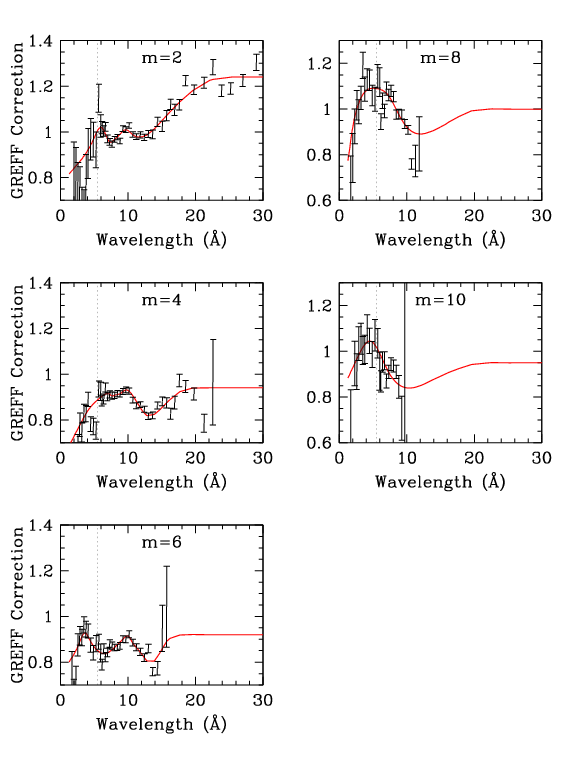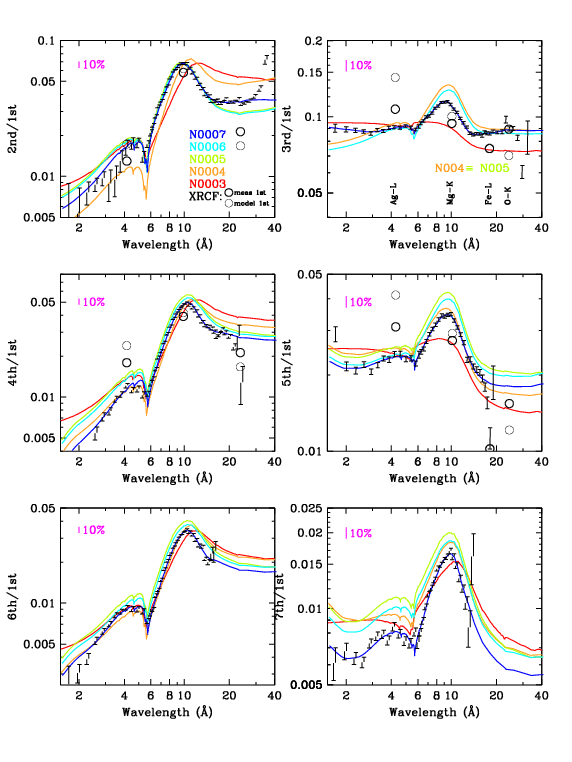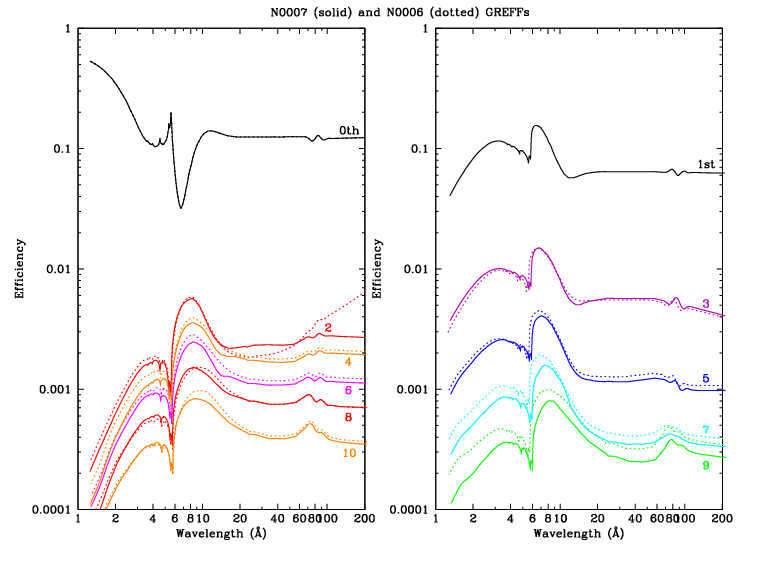Figure 1 (ps, pdf): Even orders, showing measured m/1st divided by GREFF m/1st. Here (gif, ps, pdf) is a version of the 2nd order plot that goes out to 50 Å; red circles indicate energy points in the GREFF file. Figure 2 (ps, pdf): Odd orders. The dotted vertical lines at 5.519 Å (2.247 keV) mark the Au-MV edge, where grating efficiencies change abruptly (see Figs. 3 and 4). Figure 3 (ps, pdf): Orders 2-7, comparing measured and GREFF m/1st. XRCF results are also shown (see 3rd order panel for labels), although their uncertainties may be large. Figure 4 (ps, pdf): Comparison of grating efficiencies from version N0006 and N0007. For clarity, even orders are on the left and odd orders on the right. The rise in the N0006 2nd order at long wavelengths, which dates to version N0004, was judged to be unphysical; beyond 30 Å, 2nd order is set to a constant multiple of 4th order.

GREFF Version History

N0003 was the version at launch, based on subassembly calibration and analysis of XRCF data. Further analysis of XRCF and Panter ground-calibration data led to modifications of 2nd and 3rd order in version N0004 (Jan 2001). Analysis of on-orbit LETG/ACIS data for m=2-7 generated version N0005 (Aug 2004); subsequent recognition of a systematic error caused by the spectral extraction region used in that analysis led to small reductions in the efficiencies of those same orders (2-9%, with the larger corrections for larger m) in N0006 (Apr 2010; see bottom of this page).

Improvements over the Previous (N0005) Calibration

• More high-rate long-exposure LETG/ACIS data are available,
• particularly using the standard Yoffset of +1.5', which provides a higher quality data set with a consistent set of pile-up parameters. The 2004 work had only 3 observations, each of which used a different pointing offset, making determination of pile-up parameters problematic.
• Rather than fitting peaks for each order in the ACIS energy spectra because of concerns about order sorting in some of the outer ACIS-S chips (no longer a significant concern), the number of counts as a function of order and wavelength was taken from the *pha2.fits file while the model instrument response was taken from the GARF file.
• A rectangular spectral extraction region was used, and the enclosed fraction of events for a given photon energy is virtually the same regardless of diffraction order.
• Background subtraction was handled more carefully.
• A more detailed pile-up model was used.
• More orders were studied (adding m=8, 9, 10).
• Several small details are now handled in pipeline processing such as cosmic-ray deadtime, and there have been improvements in order sorting, CTI corrections, QE uniformity calibration, gain calibration, etc.
• To reduce errors from non-linear pile-up corrections, data from time periods with especially low and high rates were excluded from Mkn421 Obsid 4148.

Data Selection and Preparation

Since higher orders are so weak, the primary criterion when choosing observations for this analysis was the total number of counts, i.e., we desired high source intensity and long exposure times. Ideally, all the observations would be made with the same pointing offsets (default Yoffset=+1.5' and Zoffset=0) and SIMZ (-8 mm) so that they would have the same line spread function in the cross-dispersion direction and therefore the same pile-up parameters. ObsID 1701 had a different Yoffset but its data were too valuable not to use (see Figs. 5 and 6); its pile-up parameters were calibrated separately. Six ObsIDs were chosen for analysis (all of those shown below except for ObsID 1703), with rates that varied over roughly a factor of 20.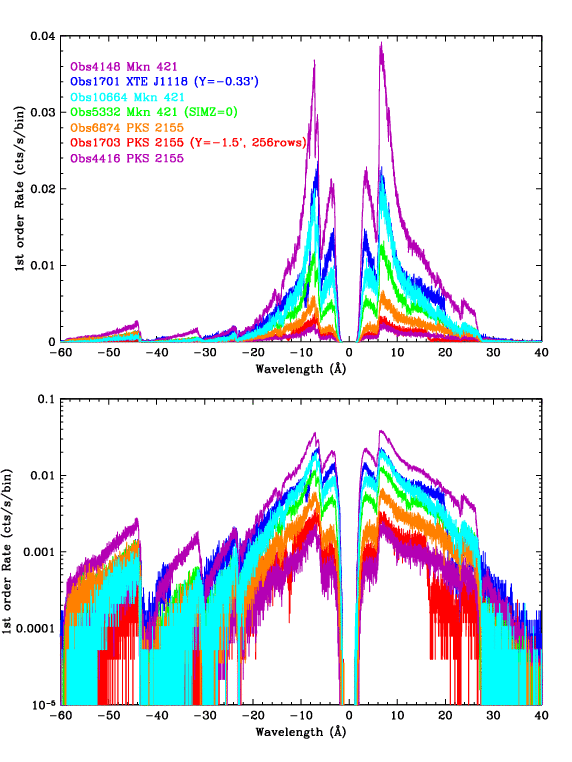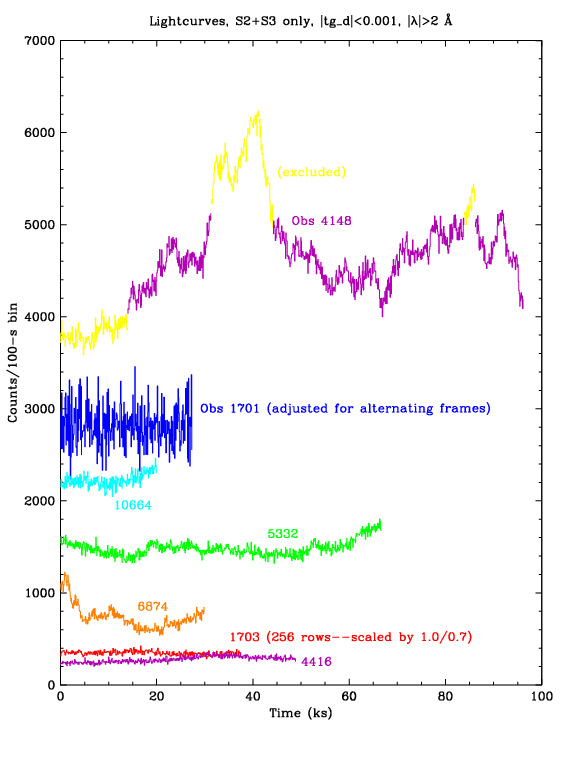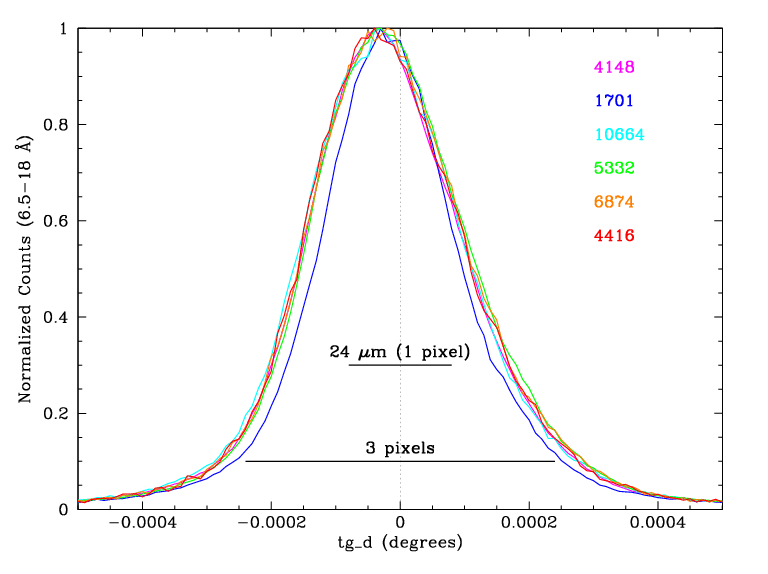Figure 5 (ps, pdf): Spectra of 7 ObsIDs, with default 0.0125-Å binning. ObsID 1701 used Yoffset=-0.33' instead of the standard +1.5' and required special tuning of its pile-up parameters because its spectrum was more tightly focused in the cross-dispersion direction (see Fig. 7). Figure 6 (ps, pdf): Spectrum light curves with 100-sec binning. Background data were also studied to remove a few periods of background flaring. Low- and high-source-rate periods of ObsID 4148 were excluded from the analysis in order to minimize nonlinearities in the pile-up corrections. ObsID 1701 used alternating-frame exposures (0.1 and 0.7 sec); only the 0.7-sec data were used. Figure 7 (ps, pdf): Cross-dispersion distributions. The profiles of the 5 ObsIDs made at Yoffset=+1.5' are virtually the same, while the distribution for ObsID 1701 (Yoffset=-0.33') is significantly narrower, requiring larger pile-up parameters.

Analysis Method

Data were reprocessed to Level 2 using the new LETG/ACIS tilt correction. All the data had severe pile-up in 0th order and downstream results at the shortest wavelengths were found to be extremely sensitive to the position of 0th order--shifts of 0.1 pixel had a significant effect--so 0th order was adjusted manually. A rectangular extraction region was used so that all orders at a given wavelength have the same extraction efficiency. A relatively wide region of |tg_d| < 0.0015 degrees was used to minimize the effects of astigmatism (see POG figures 9.14 and 9.15) at large . Background-subtracted rates were computed for each order in units of cts/frame/pixel (with distance in pixels measured along the dispersion axis) for each of 50 wavelength bins (for both + and - orders) in tg_mlam (mλ), with narrower bins in higher-count regions of the spectrum.

Pile-Up Corrections

ACIS pile-up occurs when two or more events spatially interact within a single exposure frame and are then treated as a single event. If that "single" event has a valid grade it is treated as having the summed energy of its two component events and we call it "true" pileup. If it has an invalid grade we call it "grade-migration" pileup and the event is lost. A physically based pile-up model would consider each event's charge distribution within the relevant 3x3-pixel island. Such a complex approach is impractical and instead we assume that pile-up can be approximated using only the 1-dimensional event rate of the dispersed spectrum after collapsing it in the cross-dispersion direction, effectively giving us a 3x1-pixel spatial element. Event rates are used in combination with pile-up parameters kp (for true pile-up) and kg (for grade-migration pile-up) to compute Poisson probabilities of having 0, 1, and 2 events of each kind (p or g) within the central and adjacent pixels (i.e., collapsed columns). The equations giving observed event rates (after true pile-up and grade-migration losses) are more concisely written in terms of kp and ktot (where ktot = kp +kg) and are given here.

Calibration of the Pile-Up Parameters

Pile-up parameters are different for Back-Illuminated (BI) and Front-Illuminated (FI) ACIS chips so there are 4 free parameters that must be determined. We do this by calculating the ratio of counts from different orders after applying pile-up corrections, and adjusting the pile-up parameters until good agreement is found among results from several ObsIDs with varying degrees of pile-up. Observed events from 2nd order, which has very low efficiency below ∼5.5Å, consist almost entirely of piled-up 1st order events, and so kp can be calibrated by studying the 2nd/1st ratio. Likewise, the 3rd/1st ratio from ∼6-9 Å is the most sensitive test of the value of ktot.

Count ratios (mth order divided by 1st order) are computed by first applying the pile-up corrections determined within each tg_mlam slice to each of the 0.0125/m-Å bins in the observed spectra. The appropriate GARF (or "model") is used to correct for dither across missing columns and the ratios actually used to tune the pile-up parameters and derive final results are [observed(m)/observed(1)]/[model(m)/model(1)]. Statistical errors are computed for the observed spectra and 10% (15% for ObsID 1701) of the net pileup correction is added to each order's error (simple addition, not quadrature) before finally computing the total ratio uncertainty in quadrature.

Pile-up parameters were chosen by minimizing the variance among pile-up-corrected results from the 5 ObsIDs made at Yoffset=+1.5' as pile-up parameters were varied. There is some interdependence of kp and ktot but the process was iterated and the tuning results are mostly orthogonal. Parameters for ObsID 1701 were determined by obtaining the best agreement with results from the other 5 ObsIDs.

Pile-Up-Corrected Ratios

After determining the pile-up parameters, final corrections were applied to all spectra and the error-weighted average ratios for each order were computed. Figs. 8 and 9 show the combined order-efficiency ratios before (top panels) and after (bottom) pile-up corrections.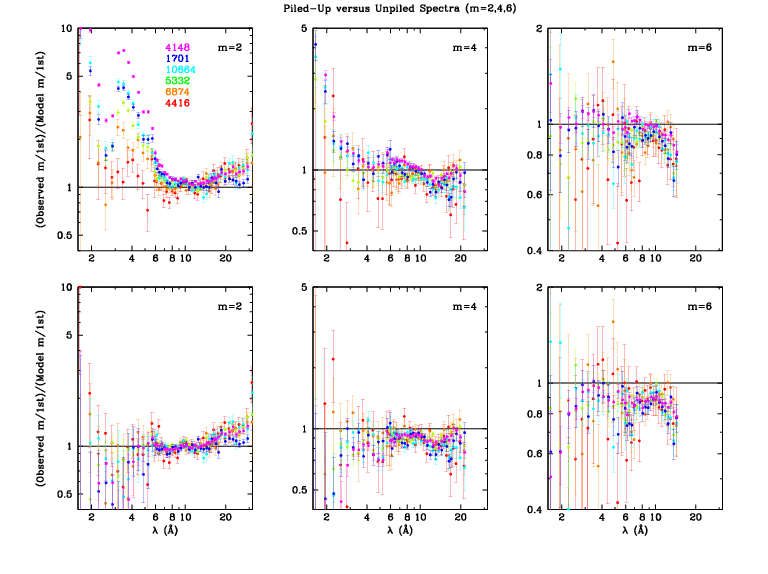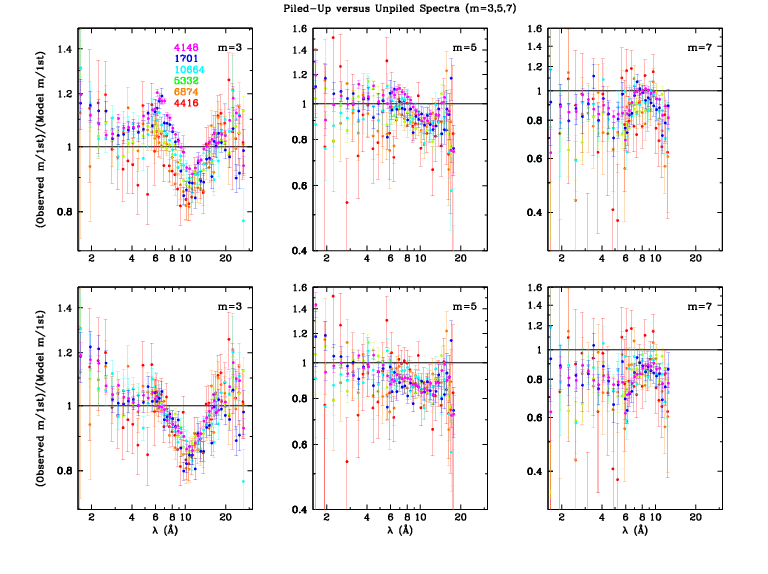Figure 8 (ps, pdf): Even orders (2,4,6) before and after pile-up corrections. Figure 9 (ps, pdf): Odd orders (3,5,7) before and after pile-up corrections.Figure 1 (ps, pdf): Even orders, showing measured m/1st divided by GREFF m/1st. Here (gif, ps, pdf) is a version of the 2nd order plot that goes out to 50 Å; red circles indicate energy points in the GREFF file. Figure 2 (ps, pdf): Odd orders. The dotted vertical lines at 5.519 Å (2.247 keV) mark the Au-MV edge, where grating efficiencies change abruptly (see Figs. 3 and 4). Figure 3 (ps, pdf): Orders 2-7, comparing measured and GREFF m/1st. XRCF results are also shown (see 3rd order panel for labels), although their uncertainties may be large. (Yes, the errorbars appear distorted but they print OK.) Figure 4 (ps, pdf): Comparison of grating efficiencies from version N0006 and N0007. For clarity, even orders are on the left and odd orders on the right. The rise in the N0006 2nd order at long wavelengths, which dates to version N0004, was judged to be unphysical; beyond 30 Å, 2nd order is set to a constant multiple of 4th order.

N0003 was the last set of GREFFs based on an analytical grating model, as opposed to the ad hoc/empirical corrections of later versions, and so we judged it to be the best theoretical guide to how grating efficiencies should behave at wavelengths beyond the LETG/ACIS useful range (roughly > 70 Å). The grating bars are effectively opaque beyond ∼30 Å, apart from some Au edge structure around 80 Å, and so grating efficiency should be constant. The rise in 2nd order efficiency beyond 30 Å, which was introduced in N0004, is therefore nonphysical and we set 2nd order to be a constant multiple of 4th order efficiency beyond that point (see Fig. 4). Similar considerations guided adjustment of other orders.

Comparison with Previous GREFFs and Significance of Changes

The general trend of the 3rd order corrections seen here matches what was seen less clearly in the 2004 N0005 analysis; no adjustment was made then because the corrections were relatively small compared to those required for other orders and because of doubts about the physical reality of the apparent deviations over such small wavelength ranges. Likewise, the relative increase in GREFF for m=2 longward of ∼15 Å (Fig. 1) was seen but not believed because of concerns over uncertainties in the ACIS calibration of the outer CCDs at such long wavelengths. With more observational data, better statistics, and other improvements in the present work, the calibration accuracy of higher orders (relative to 1st order) can now be significantly improved. The common feature of GREFF correction factors being smaller than 1 for m=4-7 is probably due to deficiencies in background subtraction in the 2004 analysis, which made the diffraction efficiencies seem larger than they were. This is particularly noticeable for m=7, which is the weakest of the orders studied in 2004.

It is unlikely that more than a few LETG observers will notice a statistically significant change in their analysis results using these new higher order efficiencies. LETG/ACIS spectra, which have order separation, should show small improvements in agreement between 1st and higher orders; 1st order fits will of course remain unchanged. More practical benefit is found for LETG/HRC spectra, which do not have enough detector energy resolution to separate orders. Strong features in line-dominated spectra will be negligibly affected, but weaker 1st order lines that blend with higher orders of stronger lines might yield significantly different fit results. For most of these weaker lines, however, statistical uncertainties, detector background, pseudo-continuum (unknown and unresolved lines), wavelength errors (multiplied by m for higher orders), and possible HRC-S distortions in line shapes and positions are likely to be larger factors than changes caused by the new higher order efficiencies.

One clear situation in which the new GREFFs will make a significant difference is when studying hard, bright spectra in a wavelength range where the 1st order spectrum is dominated by higher orders because of low-energy cutoff from interstellar absorption, for example, when looking for thermal emission in the absorbed power-law spectrum of a neutron star powering a pulsar nebula. In such situations, uncertainties in the HRC-S QE, which are larger for higher orders than for 1st order, must also be considered.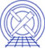The Chandra X-Ray Center (CXC) is operated for NASA by the Smithsonian Astrophysical Observatory. 60 Garden Street, Cambridge, MA 02138 USA.    Email: cxcweb@head.cfa.harvard.edu Smithsonian Institution, Copyright © 1998-2004. All rights reserved.